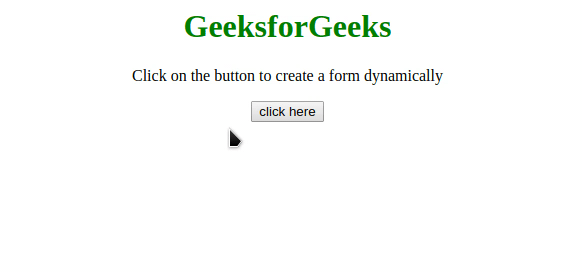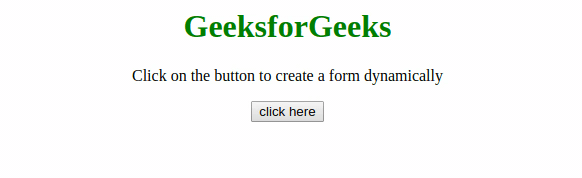Related Articles

# How to Create a Form Dynamically with the JavaScript?

• Last Updated : 30 Jul, 2021

The task is to create an HTML form dynamically with the help of JavaScript. There are two approaches that are discussed below.

Approach 1: Use document.createElement() to create the new elements and use setAttribute() method to set the attributes of elements. Append these elements to the <form> element by appendChild() method. Finally append the <form> element to the <body> element of the document. This example creates a Registration form.

• Example:
 ```<``html``>``    ``<``head``>``        ``<``title``>``            ``Create a Form Dynamically with ``            ``the JavaScript``        ````    ````    ``<``body` `style``=``"text-align: center;"``>``        ``<``h1` `style``=``"color: green;"``>``            ``GeeksforGeeks``        ````        ``<``p``>``          ``Click on the button to create``          ``a form dynamically``        ````        ``<``button` `onClick``=``"GFG_Fun()"``>``            ``click here``        ````        ``<``p` `id``=``"GFG_DOWN"``>`` ``<``script``>``    ``var down = document.getElementById("GFG_DOWN");``           ` `    ``// Create a break line element``    ``var br = document.createElement("br"); ``    ``function GFG_Fun() {``               ` `    ``// Create a form synamically``    ``var form = document.createElement("form");``    ``form.setAttribute("method", "post");``    ``form.setAttribute("action", "submit.php");`` ` `    ``// Create an input element for Full Name``    ``var FN = document.createElement("input");``    ``FN.setAttribute("type", "text");``    ``FN.setAttribute("name", "FullName");``    ``FN.setAttribute("placeholder", "Full Name");`` ` `     ``// Create an input element for date of birth``     ``var DOB = document.createElement("input");``     ``DOB.setAttribute("type", "text");``     ``DOB.setAttribute("name", "dob");``     ``DOB.setAttribute("placeholder", "DOB");`` ` `     ``// Create an input element for emailID``     ``var EID = document.createElement("input");``     ``EID.setAttribute("type", "text");``     ``EID.setAttribute("name", "emailID");``     ``EID.setAttribute("placeholder", "E-Mail ID");`` ` `      ``// Create an input element for password``      ``var PWD = document.createElement("input");``      ``PWD.setAttribute("type", "password");``      ``PWD.setAttribute("name", "password");``      ``PWD.setAttribute("placeholder", "Password");`` ` `       ``// Create an input element for retype-password``       ``var RPWD = document.createElement("input");``       ``RPWD.setAttribute("type", "password");``       ``RPWD.setAttribute("name", "reTypePassword");``       ``RPWD.setAttribute("placeholder", "ReEnter Password");`` ` `                ``// create a submit button``                ``var s = document.createElement("input");``                ``s.setAttribute("type", "submit");``                ``s.setAttribute("value", "Submit");``                 ` `                ``// Append the full name input to the form``                ``form.appendChild(FN); ``                 ` `                ``// Inserting a line break``                ``form.appendChild(br.cloneNode()); ``                 ` `                ``// Append the DOB to the form``                ``form.appendChild(DOB); ``                ``form.appendChild(br.cloneNode()); ``                 ` `                ``// Append the emailID to the form``                ``form.appendChild(EID); ``                ``form.appendChild(br.cloneNode()); ``                 ` `                ``// Append the Password to the form``                ``form.appendChild(PWD); ``                ``form.appendChild(br.cloneNode()); ``                 ` `                ``// Append the ReEnterPassword to the form``                ``form.appendChild(RPWD); ``                ``form.appendChild(br.cloneNode()); ``                 ` `                ``// Append the submit button to the form``                ``form.appendChild(s); `` ` `                ``document.getElementsByTagName("body")``               ``.appendChild(form);``            ``}``        ````    `````
• Output:Approach 2: This approach is somewhat similar to the previous one but using the JQuery method to append the elements. Use document.createElement() to create the new elements and use setAttribute() method to set the attributes of elements. Append these elements to the < form > element by append() method of JQuery. Finally append the <form> element to the <body> element of the document. This example creates a LOGIN form.

• Example:
 ```<``html``>``    ``<``head``>``        ``<``title``>``            ``Create a Form Dynamically ``            ``with the JavaScript``        ````    ````    ``<``body` `style``=``"text-align: center;"``>``        ``<``h1` `style``=``"color: green;"``>``            ``GeeksforGeeks``        ````        ``<``p``>``              ``Click on the button to create ``            ``a form dynamically``        ````        ``<``button` `onClick``=``"GFG_Fun()"``>``            ``click here``        ````        ``<``p` `id``=``"GFG_DOWN"``>``        ``<``script``>``            ``var down = document.getElementById("GFG_DOWN");``            ``function GFG_Fun() {``               ` `                ``// Create a form synamically``                ``var form = document.createElement("form");``                ``form.setAttribute("method", "post");``                ``form.setAttribute("action", "submit.php");`` ` `                ``// Create an input element for emailID``                ``var ID = document.createElement("input");``                ``ID.setAttribute("type", "text");``                ``ID.setAttribute("name", "emailID");``                ``ID.setAttribute("placeholder", "E-Mail ID");`` ` `                ``// Create an input element for password``                ``var PWD = document.createElement("input");``                ``PWD.setAttribute("type", "password");``                ``PWD.setAttribute("name", "password");``                ``PWD.setAttribute("placeholder", "Password");`` ` `                ``// Create a submit button``                ``var s = document.createElement("input");``                ``s.setAttribute("type", "submit");``                ``s.setAttribute("value", "Submit");`` ` `                ``// Append the email_ID input to the form``                ``form.append(ID); ``               ` `                ``// Append the password to the form``                ``form.append(PWD); ``               ` `                ``// Append the button to the form``                ``form.append(s); `` ` `                ``document.getElementsByTagName("body")``               ``.appendChild(form);``            ``}``        ````    `````
• Output:JavaScript is best known for web page development but it is also used in a variety of non-browser environments. You can learn JavaScript from the ground up by following this JavaScript Tutorial and JavaScript Examples.

HTML is the foundation of webpages, is used for webpage development by structuring websites and web apps.You can learn HTML from the ground up by following this HTML Tutorial and HTML Examples.

CSS is the foundation of webpages, is used for webpage development by styling websites and web apps.You can learn CSS from the ground up by following this CSS Tutorial and CSS Examples.

Attention reader! Don’t stop learning now. Get hold of all the important HTML concepts with the Web Design for Beginners | HTML  course.

My Personal Notes arrow_drop_up